# HP Forums

Full Version: Mercator Sailing: Course and Distance
You're currently viewing a stripped down version of our content. View the full version with proper formatting.
Pages: 1 2
Introduction

The following program MERCATOR calculates the course direction and distance in miles given two pairs of latitude (north/south) and longitude (east/west). Conversion to arc minutes will be required during calculation.

Code:
 EXPORT MERCATOR() BEGIN // EWS 2018-08-10 // The Calculator Afloat HAngle:=1; // degrees LOCAL L1,L2,λ1,λ2,M1,M2; LOCAL C,D; INPUT({L1,λ1,L2,λ2},"Mercator", {"L1:","λ1:","L2:","λ2:"}, {"Latitude 1", "Longitude 1", "Latitude 2", "Longitude 2"}); M1:=7915.7045*LOG(TAN(45+L1/2)) -23.2689*SIN(L1); M2:=7915.7045*LOG(TAN(45+L2/2)) -23.2689*SIN(L2); LOCAL M; C:=ABS(λ1-λ2)*60; M:=ABS(M2-M1); C:=ATAN(C/M); D:=ABS(L2-L1)*60/COS(C); RETURN {C,D}; END;

Example

Latitude 1: 102° 54’ 16” W = 102.9044444444°
Longitude 1: 43° 21’ 16” N = 43.3544444444°
Latitude 2: 106° 3’ 8” W = 106.0522222222°
Longitude 2: 42° 4’ 30” N = 42.075°

Results:

Course: 5.782390957°

Distance: 189.832588 mi

Source:

Henry H. Shufeldt and Kenneth E. Newcomer The Calculator Afloat: A Mariner’s Guide to the Electronic Calculator Naval Institute Press: Annapolis, Maryland. 1980
(08-10-2018 03:08 AM)Eddie W. Shore Wrote: [ -> ]Introduction

The following program MERCATOR calculates the course direction and distance in miles given two pairs of latitude (north/south) and longitude (east/west). Conversion to arc minutes will be required during calculation.

Source:
Henry H. Shufeldt and Kenneth E. Newcomer The Calculator Afloat: A Mariner’s Guide to the Electronic Calculator Naval Institute Press: Annapolis, Maryland. 1980
Very interesting and useful program. Do you think it could be implemented in HP 67 and/or HP 35s?
Pedro
(08-10-2018 03:08 AM)Eddie W. Shore Wrote: [ -> ]Latitude 1: 102° 54’ 16” W = 102.9044444444°
Longitude 1: 43° 21’ 16” N = 43.3544444444°
Latitude 2: 106° 3’ 8” W = 106.0522222222°
Longitude 2: 42° 4’ 30” N = 42.075°

Results:

Course: 5.782390957°

Distance: 189.832588 mi

Eddie, I think you confused latitudes and longitudes here. There are no latitudes > 90°.

I also wonder how you get these results. For the given data I get a distance of 159,03 nmi and a course of 61,14° Southwest (241,14° true course). This matches the result of an online calculator where I checked the results.

Finally, what sign convention does your program use? Are West and South positions entered with negative sign?

Dieter
Two weeks and no response. I guess Eddie is not following his own post.

I bought Calculator Afloat in the late 80s or early 90s to learn more about geodetic calculations. It's a good book, but it does not go into much detail about the signage for the latitude and longitude calculations. You perform the calculations first then mentally apply the proper signage to the results.

I modified Eddie's program where South latitudes and West longitudes must be entered as negative numbers in degrees, but I ran into a problem when the two points have the same latitude, such as

Lat 40 N, Long 25 W
Lat 40 N, Long 30 W

In this case, the course and distance equations give division by zero error messages. How do you calculate the course and distance on a parallel?

Code:
EXPORT MERCATOR() BEGIN // EWS 2018-08-10 // The Calculator Afloat HAngle:=1; // degrees LOCAL L1,L2,λ1,λ2,M1,M2; LOCAL C,D; INPUT({L1,λ1,L2,λ2},"Mercator", {"L1:","λ1:","L2:","λ2:"}, {"Latitude 1 in degrees, +N, -S", "Longitude 1 in degrees, +E, -W", "Latitude 2 in degrees, +N, -S", "Longitude 2 in degrees, +E, -W"}); M1:=7915.7045*LOG(TAN(45+L1/2)) -23.2689*SIN(L1); M2:=7915.7045*LOG(TAN(45+L2/2)) -23.2689*SIN(L2); LOCAL m,DLo,l,θ; DLo:=(λ2-λ1)*60; //difference in longitude in minutes m:=(M2-M1);      //difference in meridional parts in minutes θ:=ATAN(DLo/m);  //course in degrees relative to North (+ or -) or South (+ or -) l:=(L2-L1)*60;   //difference in latitude in minutes IF DLo>=0 AND m>=0 THEN   C:=θ; END; IF DLo<0 AND m>=0 THEN    C:=360+θ;  END; IF DLo<0 AND m<0 THEN   C:= 180+θ; END; IF DLo>=0 AND m<0 THEN   C:=180+θ; END; D:=ABS(l/COS(θ)); RETURN {C,D}; END;
(08-24-2018 07:21 PM)Gene222 Wrote: [ -> ]I modified Eddie's program where South latitudes and West longitudes must be entered as negative numbers in degrees, but I ran into a problem when the two points have the same latitude, such as

Lat 40 N, Long 25 W
Lat 40 N, Long 30 W

In this case, the course and distance equations give division by zero error messages. How do you calculate the course and distance on a parallel?

I wrote a Mercator Saling program for the 35s and encountered the same problem. However, a bit of calculus yields the following result:

Code:
                                           1 - e²·sin²(lat) distance = 60·|long2 - long1| · cos(lat) · ----------------                                                 1 - e²

The course c then is 0° (exactly East or West). Or 90° / 270° true course.

Here "e" is the eccentricity of the assumed ellipsoid (around 0,082). This is not explicitely considered in the approximate formula given in the book (as used in Namir's program).

But we can do better: calculate the meridional parts (M) as follows using hyperbolic functions:

Code:
M = 60·180/pi · [artanh(sin(lat)) – e·artanh(e·sin(lat))]

You can also do it without hyperbolics:

Code:
M = 60·180/pi · ln[tan(45° + lat/2) · ((1–e·sin(lat))/(1+e·sin(lat))^(e/2)]

BTW, the constant 7915,7045 in the approximate formula is 60·180/pi · ln10 due to the use of the base 10 logarithm instead of the natural log.

Both formulas are more accurate and allow implementing different ellipsoids. I prefer the one with the two artanhs since it is more compact and ...elegant. ;-)

For "e" you may use these values:
Clarke 1880 ellipsoid: e = 0,082483399
WGS84 / GRS80 ellipsoid: e = 0,081819191

For your example the distance is 230,7 nmi (for both ellipsoids the results differ by less than 0,02 nmi).

Check: use your program "as is" and make the latitudes slightly different, e.g. 40° and 40,0001°. This should give (nearly) the same result.

Remark: instead of calculating the arctan you can determine the angle with a R→P conversion (automatically selects the correct quadrant) followed by mod 360° to get a positive angle. This way the four IF cases are not required (BTW, are you sure the last two are both correct?).

Dieter
"a bit of calculus"??? Your math skills are far better than mine. It looks like you are calculating the distance directly from the ellipsoid and not from the map projection. I did remember of another way to solve the course and distance on an East-West line for pt 1 and pt 2. This was on a old forum. This person said to create a third point mid-longitude between the two points, but with a latitude well above the two points. Then calculate the course and distance from pt 1 to pt 3 and from pt 3 to pt 2. He then added the vectors to give him the course and distance from pt 1 to pt 2. I need to think about this East-West issue some more.

Regarding the WGS84 ellipsoid, it certainly makes sense to use a more modern ellipsoid that is used by GPS, but this is a Mercator Sailing program. The WGS84 ellipsoid seem more like Rhumb-line sailing. Regarding the accuracy of the author's equation, I did find a more accurate meridian part equation from an on-line Mercator calculator's site. I don't know if it is Clark's ellipsoid of 1866, but it closely resembles the author's equation.

Calculator Afloat equation
Meridinal Part 1 = 7915.7045*LOG(TAN(45+Lat1/2))-23.2689*SIN(Lat1);

Online Mercator equation
Meridinal Part 1 = 7915.704468*LOG(TAN(45+Lat1/2))-23.268932*SIN(Lat1)-0.052500*(sin(Lat1))^3-0.000213*(sin(Lat1))^5;

I think I need to keep this program simple and stick with the Mercator sailing equations. I can add a more user friendly input and provided a output that give some intermediate results in addition to the course and distance. I can also adjust the program to handle equatorial crossings (m=M1+M2, but adjusted for direction) and 180 deg meridian crossings. I not sure what I will do about East-West courses. But first, I will take a break from programming and maybe read more on Rhumb-line sailing.
(08-26-2018 12:30 AM)Gene222 Wrote: [ -> ]"a bit of calculus"??? Your math skills are far better than mine.

It's just the limit for the distance as lat1 → lat2.
Re. math skills: the result includes the derivative M'(lat). I was lazy and had it calculated by Wolfram Alpha. ;-)

(08-26-2018 12:30 AM)Gene222 Wrote: [ -> ]It looks like you are calculating the distance directly from the ellipsoid and not from the map projection.

No, these are the Mercator sailing equations. The meridional parts calculation is just a bit more accurate in that the eccentricity e is not hidden in other constants so that it can be adjusted. Compare the results with other sources, e.g. online calculators.

Hint: the expression ln(tan(45°+x/2)) in the "book" formula is equivalent to artanh(sin(x)).

For a course on the same parallel the given formula simply calculates the limit of the original formula as the two latitudes approach each other. Try it: use the original formula and make the two latitudes almost equal, e.g. 40° and 40,0001°. You will get the same result.

(08-26-2018 12:30 AM)Gene222 Wrote: [ -> ]I did remember of another way to solve the course and distance on an East-West line for pt 1 and pt 2. This was on a old forum. This person said to create a third point mid-longitude between the two points, but with a latitude well above the two points. Then calculate the course and distance from pt 1 to pt 3 and from pt 3 to pt 2. He then added the vectors to give him the course and distance from pt 1 to pt 2. I need to think about this East-West issue some more.

Way too complicated. The above formula works.

(08-26-2018 12:30 AM)Gene222 Wrote: [ -> ]Regarding the WGS84 ellipsoid, it certainly makes sense to use a more modern ellipsoid that is used by GPS, but this is a Mercator Sailing program. The WGS84 ellipsoid seem more like Rhumb-line sailing. Regarding the accuracy of the author's equation, I did find a more accurate meridian part equation from an on-line Mercator calculator's site. I don't know if it is Clark's ellipsoid of 1866, but it closely resembles the author's equation.

That looks like a series expansion of the true formula.
Of course you can also use this approximation. But why do so if you can have the exact result?

(08-26-2018 12:30 AM)Gene222 Wrote: [ -> ]I think I need to keep this program simple and stick with the Mercator sailing equations.

Again: these are the Mercator sailing equations.
And the artanh method makes them even more compact.

Gene, it looks like you think that the formulas I gave calculate something different from the Mercator sailing course and distance. They don't. The Meridional parts formula is just more accurate and can handle different ellipsoids. The results match exactly what is shown in other online sources on this topic. And the East-West-formula is exactly what you get with the original formula if the two latitudes approach each other.

Edit:
Maybe a few examples are more convincing. Please take a look at this PDF with some calculations including the meridional parts. I assume their values were taken from nautical tables, so they may serve as a reference. Here are the values from the PDF, then those calculated with the artanh formula (assuming the Clarke ellipsoid) and finally using the simplified formula due to "The Calculator Afloat".

Code:
 given                     Meridional Parts latitude      due to PDF    artanh formula    simple formula ------------------------------------------------------------  42°45'        2826,73          2826,73          2826,83  27°30'        1706,46          1706,46          1706,52  47°14,3'      3206,54          3206,54          3206,64  43°55'        2922,63          2922,63          2922,73

You see that the artanh formula exactly matches the values in the PDF (which I assume are correct) while the results from the formula in the book are slightly high (~ +0,1). The latter doesn't matter much as in our case only the difference between the meridional parts of the two positions is considered. Also an accuracy of one decimal seems to be adequate here.

BTW, you can minimize the error of the simple formula if you replace the constant 23,2689 with 23,4286 for the Clarke ellipsoid or with 23,0522 for WGS84/GRS80. This way the deviation is less than ±0,014 meridional parts for any latitude. On the other hand 23,2689 is the best possible value for an eccentricity of 0,0822... which is roughly in the middle between the two mentioned ellipsoids.

But instead of all this you can also simply use the more accurate formula. Either the artanh version or the other one. ;-)

Edit 2:
If you want to stick to the simplified formula you can determine the distance on the same parallel (lat) as follows:

Code:
                                                     1 distance  =  120 · |long2 - long1| / [ ----------------------------- – 23,2689·pi/5400·cos(lat) ]                                        sin(45°+lat/2)·cos(45°+lat/2)
The constant in the last term can be simplified to 0,0135373. Or, even shorter, 1/73,87.

For your example this evaluates to 230,73 nmi.

Dieter
Regarding the equation for meridional parts, I saw a August 2012 forum on gcaptain.com. Students were discussing how to solve a Mercator Sailing problem with the moderators in preparation for their maritime license exam. What was interesting was that they were calculating the merdional parts using table 6 in Bowditch. They said that in the explanation of the merdional parts tables in the 1975 edition of Bowditch, the meridional parts were based on the Clarke spheroid of 1866, while in the 2002 edition of Bowditch, the meridional parts were based on the WGS ellipsoid of 1972. What I got out of this was that Bowditch was an authoritative reference on determining the merdional parts for Mercator Sailing and it explains how the meridional parts are calculated.

Bowditch is short for American Practical Navigator, originally by Nathanial Bowditch and published by the National Geospatial-Intelligence Agency, Springfield, Virginia. Bowditch was first published in 1802. On page 2 of Volume 2 of the 2017 edition of Bowditch, the formula to calculate the merdional parts is

M = a ln(10) log tan (45 + L/2) - a (e^2 sin L + e^4 / 3 sin^3 L + e^6/5 sin^5 L ...

(You really need to see this in textbook mode to make sense of it)

a is the equitorial radius of the earth expressed in minutes of arc of the equator = 3437.74677078.

ln(10) is 2.3025851

L is the latitude

f is earth's flattening = 3.35281066475*10^(-3)

e^2 is 2f - f^2 = 6.694379990141*10^(-3)

where the constants are based upon the World Geodetic System 1984 (WGS84). Plugging in the constants, they show

M = 7915.7 log tan(45deg + L/2) - 23.01358319 sin L - 0.05135389 sin^3 L - 0.00020627 sin^5 L ...

For reasons unknown, they rounded the first constant. If you multiply a ln(10) using their numbers, you get

M = 7915.70446787 log tan(45deg + L/2) - 23.01358319 sin L - 0.05135389 sin^3 L - 0.00020627 sin^5 L ...

This is the formula that should be used to calculate the merdional parts for Mercator Sailing, and it is based on the WGS84 ellipsoid. I never seen the expression - 0.05135389 sin^3 L. Is that the same -0.05135389 sin (L)^3 or -(0.05135389 sin L)^3?
(08-28-2018 12:46 AM)Gene222 Wrote: [ -> ]M = a ln(10) log tan (45 + L/2) - a (e^2 sin L + e^4 / 3 sin^3 L + e^6/5 sin^5 L ...

Thank you very much for this formula.

(08-28-2018 12:46 AM)Gene222 Wrote: [ -> ]a is the equitorial radius of the earth expressed in minutes of arc of the equator = 3437.74677078.

This is the factor 60*180/pi in my formula.
Circumference at equator in arc minutes = 60 · 360° = 21600'
Radius at equator = circumference / 2$$\pi$$ = 60 · 180 / $$\pi$$ = 3437,7467707849392526...

Now let's compare the Bowditch formula with the one I posted, assuming e=0,081819191 for WGS84.
The Bowditch formula was used with exact (12-digit) coefficients and one more term.

L=5°
Bowditch: 298,375698376
Artanh:   298,375698374

L=10°
Bowditch: 599,073043655
Artanh:   599,073043671

L=20°
Bowditch: 1217,26588915
Artanh:   1217,26588917

L=30°
Bowditch: 1876,86220651
Artanh:   1876,86220652

L=40°
Bowditch: 2607,88368513
Artanh:   2607,88368514

L=50°
Bowditch: 3456,82030073
Artanh:   3456,82030073

L=60°
Bowditch: 4507,40395347
Artanh:   4507,40395348

L=70°
Bowditch: 5944,24941337
Artanh:   5944,24941337

L=80°
Bowditch: 8352,48380806
Artanh:   8352,48380805

L=85°
Bowditch: 10741,6440576
Artanh:   10741,6440579

L=87°
Bowditch: 12499,0738936
Artanh:   12499,0738943

L=89°
Bowditch: 16276,4947743
Artanh:   16276,4947699

Convinced ?-)

I think we can safely assume that the slight differences beyond the 10th digit are due to roundoff errors, especially as L approaches 90°. That's why with 12-digit precision the 89° artanh result is slightly off. The 34s here returns 16276,49477437... ;-)

Edit:
I have now compared both formulas in Excel (15 digits). The more terms you add to the Bowditch formula the closer it matches the result of the artanh formula. With terms up to sin(L)^11 and exact coefficients the results agree within a few ULP. So the Bowditch formula seems to be a series expansion of the artanh formula. The latter essentially has two advantages: you don't need half a dozen of numeric constants but just one, the eccentricity e, and the whole thing is much shorter.

Dieter
(08-28-2018 08:53 AM)Dieter Wrote: [ -> ]I have now compared both formulas in Excel (15 digits). The more terms you add to the Bowditch formula the closer it matches the result of the artanh formula. With terms up to sin(L)^11 and exact coefficients the results agree within a few ULP. So the Bowditch formula seems to be a series expansion of the artanh formula.

Indeed, this is actually is the case here:

e2 sin(L) + e4 sin3(L)/3 + e6 sin5(L)/5 + e8 sin7(L)/7 + ...  =  e · artanh(e · sin(L))

7915,7044678978...· lg(tan(45°+L/2))  =  60·180/$$\pi$$ · ln(tan(45°+L/2))  =  60·180/$$\pi$$ · artanh(sin(L))  =  60·180/$$\pi$$ · arsinh(tan(L))

Note: the 12-digit value of the first constant is 7915,70446790 and not ...87

In other words: the Bowditch formula and the artanh version I posted are mathematically equivalent. They give the same results. The artanh formula just is much more compact and requires merely one single constant, the eccentricity e. I like it. ;-)

BTW, close to 90° (where Mercator's projection is problematic anyway) the original formula loses some accuracy. But this can be improved by modifying the formula slightly:

M  =  60·180/$$\pi$$ · [arsinh(tan(lat)) – e·artanh(e·sin(lat))]

Dieter
Okay. You convinced me. I will use your ATANH formula (the first one). The formula really does need to be accurate to transition to the formula for the East-West course. I think I got the program just about done, but I need to recheck it to see if I got the signage right for all cases. I got hung up on the 0 and 180 degree meridian crossings. Attached are the Mercator Sailing signage rules that were not included in the Calculator AFloat book.

EDIT 11/9/18. The following is from The Yachtsman’s Handybook by WH Rosser, Charles Wilson Publisher, 1877, Brief Rules In Navigation Chapter, 6: Course and Distance by Mercator’s Sailing Section.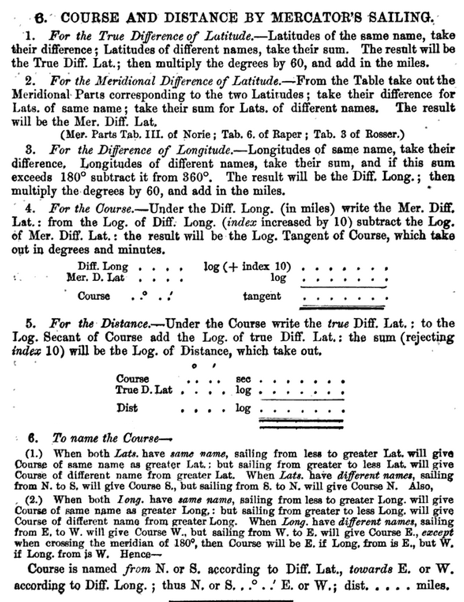(08-29-2018 09:02 PM)Gene222 Wrote: [ -> ]Okay. You convinced me. I will use your ATANH formula (the first one). The formula really does need to be accurate to transition to the formula for the East-West course.

It is. For lat1 = lat 2 use the formula from post #5:

Code:
                                           1 – e²·sin²(lat) distance = 60·|long2 – long1| · cos(lat) · ----------------                                                 1 – e²

Check it yourself: calculate the distance for two almost identical latitudes (say, 40 and 40,00001 degrees) and compare the result with the above formula.

(08-29-2018 09:02 PM)Gene222 Wrote: [ -> ]I think I got the program just about done, but I need to recheck it to see if I got the signage right for all cases. I got hung up on the 0 and 180 degree meridian crossings.

Make sure the absolute value of long2 – long1 does not exceed 180°. For example, 250° East is equivalent to 110° West. Continue with this longitude difference.

Dieter
I am still hung up on 180 meridian crossings, or maybe not. I ran my program to calculate the course and distance of a 180 degree meridian polar crossing.
Pt1: Lat 80 N, Long 45 E
Pt2: Lat 80 N, Long 135 W
The program gave me a course of 270 deg and a distance of 1875.7814 nm, which does not make any sense to me. I inputted the same coordinates on the starpath.com Mercator Calculator, and it gave a course of 270 deg and a distance of 1875.4003 nm, which also makes no sense to me. After thinking about it, I am beginning to think Mercator Sailing simply can't handle a 180 deg polar crossings. What do you think? If it is not a limitation of Mercator Sailing, I don't think I am interested in fixing this. My program is attached.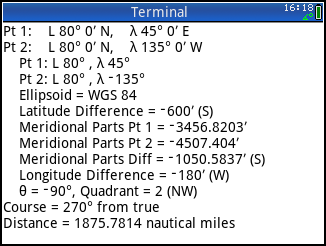(08-30-2018 11:59 PM)Gene222 Wrote: [ -> ]I am still hung up on 180 meridian crossings, or maybe not. I ran my program to calculate the course and distance of a 180 degree meridian polar crossing.
Pt1: Lat 80 N, Long 45 E
Pt2: Lat 80 N, Long 135 W
The program gave me a course of 270 deg and a distance of 1875.7814 nm, which does not make any sense to me.

I see what I am doing wrong. The course is 270 (due west) on a Mercator map. I was looking a polar map. I guess starpath.com online Mercator calculator was right. EDIT 8/30/18. The path on the Mercator map is a straight line at 270 degrees. The path on a polar map is a semi-circle. I guess this is why they have Great Circle Sailing. I still have errors in my program. The Latitude difference for the above problem is 600' and the Meridional parts for point 1 and 2 are not the same. My procedure for East-West courses needs to be cleaned up, which should be easy to do. Lastly, I am using an eccentricity of 0.081819190842622 for the WGS84 ellipsoid from the Department of Defense World Geodetic System 1984, National Imagery and Mapping Agency, 3rd Ed, Amendment 1, Jan 2000.
(08-31-2018 02:15 AM)Gene222 Wrote: [ -> ]I see what I am doing wrong. The course is 270 (due west) on a Mercator map. I was looking a polar map. I guess starpath.com online Mercator calculator was right.

For the record: my 35s program returns 1875,78 miles and a course exactly West, true course 270°.

By the way, the result 1875,4003 nm of the starpath.com Mercator Calculator simply is 180°·60·cos(80°). This is a simplified formula that ignores (!) the ellipsoid's eccentricity. That's why there is another factor in my same-latitude-formula – which returns the correct result.

(08-31-2018 02:15 AM)Gene222 Wrote: [ -> ]I still have errors in my program. The Latitude difference for the above problem is 600' and the Meridional parts for point 1 and 2 are not the same.

This really sounds like there are errors. ;-)
For 80° the meridional parts (WGS84) should be 8352,48.

Your results of –3456,8203 and –4507,4040 are the meridional part values for latitudes of –50° and –60°, respectively. Maybe this helps finding the error.

(08-31-2018 02:15 AM)Gene222 Wrote: [ -> ]My procedure for East-West courses needs to be cleaned up, which should be easy to do.

I can't read your program (no Prime here), but from what I see when I open it in a text editor the program distinguishes various cases for different hemispheres. For instance in one case the absolute values of the meridional parts are added. I don't think this is required – the sign convention handles this automatically. Try it.

Here is the way I do the calculation, in pseudocode. I hope I got it right. ;-)

Code:
e=0,08181919... M1=Meridionalparts(lat1)     M2=Meridionalparts(lat2) DMP=M2-M1 dlong=long2-long1 if dlong < -180 then dlong=dlong+360 if dlong > +180 then dlong=dlong-360    // edit: corrected this line dlongminutes=60*dlong dlatminutes=60*(lat2-lat1) if DMP=0 then    course=0    distance=abs(dlongminutes)*cos(lat1)*(1-e^2*sin^2(lat1))/(1-e^2) else    course=arctan(abs(dlongminutes/DMP))    distance=abs(dlatminutes)/cos(course) end

Finally the true course is calculated. I do it wih the 35s "ARG" command which returns something like ATAN2 in some programming languages, a quadrant-adjusted angle (0...180 for Q1 and Q2, 0...-180 for Q3 and Q4, in this case add 360°), and it also works for DMP=0. You'll know how to do it on the Prime. ;-)

Dieter
Deleted by user.
(08-31-2018 05:46 AM)Dieter Wrote: [ -> ]Here is the way I do the calculation, in pseudocode. I hope I got it right. ;-)

There was an error in the adjustment of the longitude difference dlong, I have corrected this now.

Gene: could you post the source code of your program, please? I can't open .hpprgm files.

Dieter
I incorporated most of the changes you suggested. Attached is the revised program.

Example Problem. Our position is L 32°14.703′ N, λ 66°28.902′ W, and we wish to determine the course and distance to a point near Chesapeake Light, in L 36°58.703′ N, λ 75°42.201′ W, by Mercator sailing.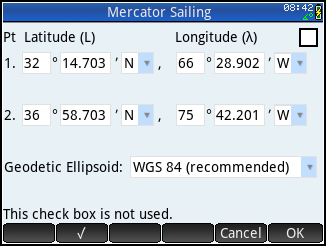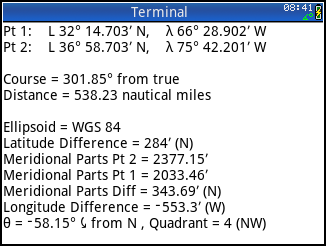(09-02-2018 04:03 PM)Gene222 Wrote: [ -> ]I incorporated most of the changes you suggested. Attached is the revised program.

Looks very good now – thumbs up. ;-)

I tried to read the program by removing the header and trailer portion of the .hpprgm file and then delete all null bytes. And I'd suggest two changes:

– The allowed latitude range is 0 ≤ L < 90°. So an input of 90° is not permitted. This can be handled by a few simple changes in the respective tests. More precisely, the largest latitude is ~89,99994° as sin(89,99995°) already rounds to 1 and artanh(1) is not defined. So this is the limit. You may simply check if sin(lat)=1. Or maybe you should limit the latitude to something more sensible like 89° or even 85° which seems more adequate for maps with Mercator projection. This also addresses the reduced accuracy issue of the original artanh(sin) formula close to 90°.

– Instead of checking whether lat1 = lat2 I think it's safer to compare the corresponding meridional parts, i.e. check if MeridPartDiff=0. You never know when roundoff errors may occur. ;-) However, if the latitude minute input is limited to 3 decimals (don't know how the Prime behaves here) no change is required.

Edit: I just noticed this line in the East-West portion of the code: BearingQuad:="2 (NW)"; Shouldn't this be "4 (NW)"?
And in the distance formula the two constants "60" cancel out and thus can be removed.

Finally: what about one or two examples for an exact East-West or West-East course?

Dieter
Thanks for your comments on the program. I definitely bit off more than I could chew with this program. The revised program is attached.

Regarding the header and footer text that can be seen when the program is viewed as text file, I had previously copied the hp program code into WordPad 6.3 for Windows 8.1. I used WordPad's find and replace to change some of the variable names. I then copied the code back into the hp prime connectivity kit program editor. I had no idea that the pasted text from WordPad included all that gobbledygook information in the header and footer of the program. Some of the information is the revision history of the find and replace operations. I do not understand what null bytes are or what this gobbledygook information really is, so I just left it in the hp prime program.

I changed the upper limit of the latitude to be 85 degrees. A latitude of 85 degrees includes all of Greenland and the antarctic seas. The Meridional Parts table 6 in Bowditch goes up to 89°59′ (MP=30351.6), but you really should not be using Mercator Sailing in the polar regions. The book Calculator Afloat says you should use a different method such as using both Rhumb-line and Great Circle sailing in tandem. Bowditch says that for the polar regions, you should use a local conic projection map of the area or an Oblique Mercator Map where the Earth is tilted about 45 degrees so that the north pole appears in the mid-northern latitudes or some other map projection. I modified the program to print a note that this program is not for polar regions and is only for educational use. The program was designed to print out the intermediate results as if one were calculating the course and distance by hand using a Meridional Parts table.

I changed the program to check for the MeridPartDiff=0 instead of Lat1=Lat2.

I rewrote the procedure for East-West lines to check for all four cases (NE, SE, SW, and NW). The procedure seems to also work with 0 and 180 meridian crossings.

I think I got all the errors corrected, but I said that before.

Example 1. Our position is L 32°14.703′ N, λ 66°28.902′ W, and we wish to determine the course and distance to a point near Chesapeake Light, in L 36°58.703′ N, λ 75°42.201′ W, by Mercator sailing.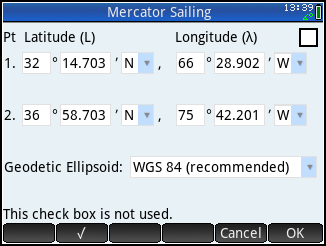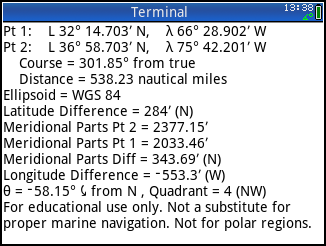Example 2 for an East-West course. Our position is a point off Port Aransas, Corpus Christi, Texas at L 27°50.1′ N, λ 97°0.5′ W, determine the course and distance to a point north of Tampa Bay, Florida at L 27°50.1′ N, λ 82°58.1′ W.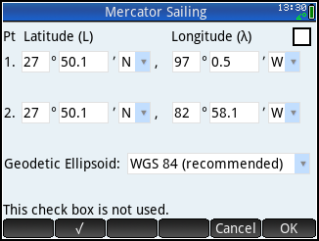Pages: 1 2
Reference URL's
• HP Forums: https://www.hpmuseum.org/forum/index.php
• :